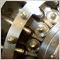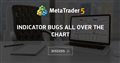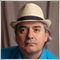# Set the bars in calculation - page 211694

Keith Watford:
IndicatorCounted() is an outdated function and it is probably a good idea to stop using it and do all calculations with rates_total and prev_calculated.

I said that IndicatorCounted() is working ~ It's the latest standard which I have problems with. Can you understand that I have not seen prev_calculated equal anything but 0? Maybe you see something different was what I was wondering. No it's MQL4 but it's an indicator same as any indicator I will currently find in technical indicators.Moderator
32146

prev_calculated will equal 0 when the indicator receives its first tick.
After that it will equal rates_total from the previous tick unless more than 1 bar is updated at the same time, in which case it will equal 0 for that 1 tick. Very useful as you can be sure that the indicator can't miss bars.

What are you returning from OnCalculate()? Have you changed it from the default so that it is returning 0? If so, that is why you are having problems.

No it's MQL4 but it's an indicator same as any indicator I will currently find in technical indicators.

There is a reason that MQL4 has its own section and that is because MQL4 indicators are not the same as MQL5 indicators. Ie. MQL5 does not have the function IndicatorCounted().

When you post in the wrong section it can cause confusion. It can also waste people's time when they take time to reply thinking that the subject is MQL5.

So, in future, please post anything concerning MQL4 in this section.11694

Keith Watford:Indicator bugs all over the Chart
• 2021.01.25
• www.mql5.com
Hello Community I got a little problem with a Indicator I made...6954

Brian Lillard: Can you understand that I have not seen prev_calculated equal anything but 0? Maybe you see something different was what I was wondering.

Don't use "IndicatorCounted()", not even in MQL4. Its obsolete!

The reason that "prev_calculated" is always 0 is because you are not returning any value from within the OnCalculate() event handler. Normally you would return the "rates_total" once you have processed everything. Please read the documentation next time.

Using your posted code as reference:

```int OnCalculate(const int rates_total,const int prev_calculated,const datetime &time[],
const double &open[],const double &high[],const double &low[],const double &close[],
const long &tick_volume[],const long &volume[],const int &spread[])
{
int iIndicatorCounted=IndicatorCounted();
int iPrevCalculated=prev_calculated;

Comment(
"IndicatorCounted(): "+(string)iIndicatorCounted+"\n"+
"BarsInCalc & IndicatorCounted(): "+(string)(BarsInCalc-(iIndicatorCounted>0?BarsInCalc:iIndicatorCounted))+"\n"+
"rates_total-prev_calculated: "+(string)(rates_total-prev_calculated)
);

return rates_total; // You are missing a return value in order for the next "prev_calculated" to update.
}```11694

Fernando Carreiro:

Don't use "IndicatorCounted()", not even in MQL4. Its obsolete!

The reason that "prev_calculated" is always 0 is because you are not returning any value from within the OnCalculate() event handler. Normally you would return the "rates_total" once you have processed everything. Please read the documentation next time.

Using your posted code as reference:

way to go guys having a laugh at my expense

let me say I am not too happy with either of your assistance ;))6954

Brian Lillard: way to go guys having a laugh at my expense let me say I am not too happy with either of your assistance ;))

What on earth are you talking about? I did not disrespect or belittle you, and I gave you an explanation of the problem and a solution to help you.

Fine. If that is your attitude, then I will make sure never to offer any help to you in the future, so as not to get such an ungrateful response!Moderator
32146

Brian Lillard:

way to go guys having a laugh at my expense

let me say I am not too happy with either of your assistance ;))

How are we "having a laugh at your expense"?

Both Fernando and I have basically said the same thing which was intended so that you would know why prev_calculated was always equal to 0.

We are trying to help, but you're not happy with that??Moderator
32146

Why have you linked to an earlier post of mine?

Is it meant to mean something?11694

Hmm? Did any of you offer any solution here? I have been trying to get my point across from the beginning of this thread and it will serve me to remember both of your behavior in the future as rude and cumbersome. Not to say that not just anybody could come along and be wrong but that you are pushing something that makes no sense - it doesn't really matter what you think anymore compared to someone else with just the wrong answers. I think I can do without this behavior and stress! No solution bro I have not seen prev_calculated ever be anything but 0 and the solution would had been obviously to incorporate a bars in calculation rather than rates_total I demonstrated it even in a gif and get that I didn't have a return in the example as if that's a reason for anything like I could have compiled without it to take that screenshot. Please! You could expect that you will be getting any greatful response. Real talk you got something against me so don't come bullying me again it's just that simple. I thinkModerator
32146

For other people who may read this topic.
Brian is completely wrong when he says that prev_calculated always equals 0.

Fernando and I have both given him the reason why this is happening for him,

I wrote very simple code to check.

```int OnCalculate(const int rates_total,
const int prev_calculated,
const datetime &time[],
const double &open[],
const double &high[],
const double &low[],
const double &close[],
const long &tick_volume[],
const long &volume[],
{
Print("prev_calculated = ",prev_calculated);

//--- return value of prev_calculated for next call
return(rates_total);
}```

and put it on a M1 chart. The result.

0 18:10:12.586 Custom indicator ####prev_calculated GBPUSD-g,M1: loaded successfully

0 18:10:14.550 ####prev_calculated GBPUSD-g,M1: initialized

0 18:10:14.550 ####prev_calculated GBPUSD-g,M1: prev_calculated = 0

0 18:10:24.599 ####prev_calculated GBPUSD-g,M1: prev_calculated = 2049

0 18:10:29.494 ####prev_calculated GBPUSD-g,M1: prev_calculated = 2049

0 18:10:29.735 ####prev_calculated GBPUSD-g,M1: prev_calculated = 2049

0 18:10:40.792 ####prev_calculated GBPUSD-g,M1: prev_calculated = 2049

0 18:10:41.811 ####prev_calculated GBPUSD-g,M1: prev_calculated = 2049

0 18:10:41.829 ####prev_calculated GBPUSD-g,M1: prev_calculated = 2049

0 18:10:41.888 ####prev_calculated GBPUSD-g,M1: prev_calculated = 2049

0 18:10:43.724 ####prev_calculated GBPUSD-g,M1: prev_calculated = 2049

0 18:10:43.802 ####prev_calculated GBPUSD-g,M1: prev_calculated = 2049

0 18:10:47.318 ####prev_calculated GBPUSD-g,M1: prev_calculated = 2049

0 18:10:50.663 ####prev_calculated GBPUSD-g,M1: prev_calculated = 2049

0 18:10:50.748 ####prev_calculated GBPUSD-g,M1: prev_calculated = 2050

0 18:10:50.895 ####prev_calculated GBPUSD-g,M1: prev_calculated = 2050

0 18:10:51.169 ####prev_calculated GBPUSD-g,M1: prev_calculated = 2050

0 18:10:51.663 ####prev_calculated GBPUSD-g,M1: prev_calculated = 2050

0 18:10:52.222 ####prev_calculated GBPUSD-g,M1: prev_calculated = 2050

0 18:10:53.013 ####prev_calculated GBPUSD-g,M1: prev_calculated = 2050

0 18:10:53.143 ####prev_calculated GBPUSD-g,M1: prev_calculated = 2050

0 18:10:59.989 ####prev_calculated GBPUSD-g,M1: prev_calculated = 2050

0 18:11:00.157 ####prev_calculated GBPUSD-g,M1: prev_calculated = 2050

Now as you can see, the first time OnCalculate() is called, prev_calculated = 0.

The next call it is 2049 and after the first tick of a new bar it is 2050.

So it definitely does not always equal 0.

I don't know why Fernando and I have been accused of bullying and being wrong as we have simply stated where he is going wrong.

No bullying, just helping.

Neither of us deserve that unwarranted response.